# SSAT Upper Level Math : How to graph a point

## Example Questions

← Previous 1

### Example Question #1 : How To Graph A Point

What are the coordinates of point B?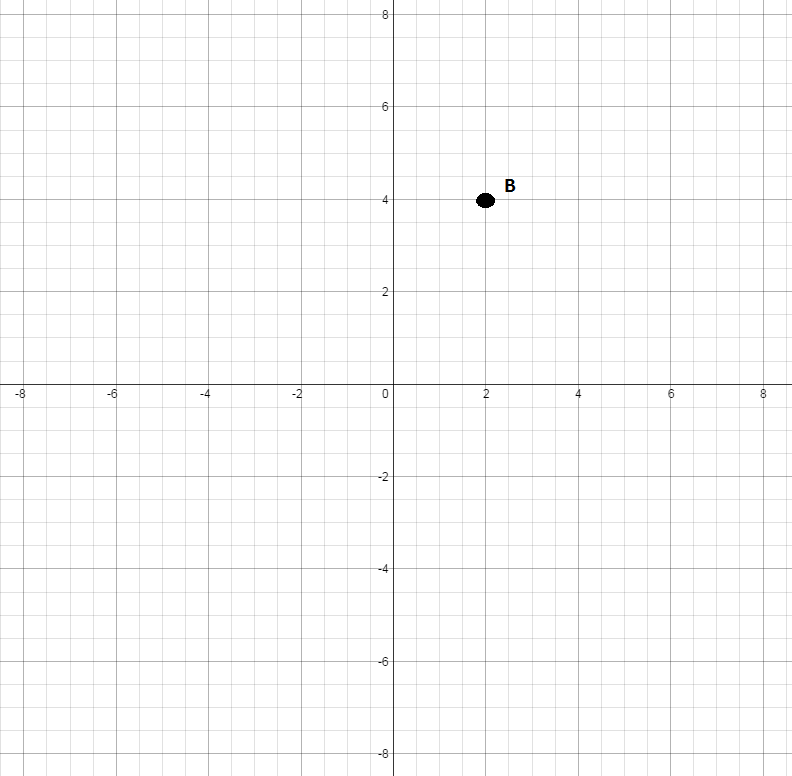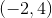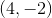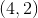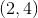Explanation:

Find the x-coordinate by going right on the horizontal x-axis until you come across the line that is directly under the point. The x-coordinate is.

Now, continue up until you reach the point and look across to the vertical y-axis. The y-coordinate is.is then the coordinate of the point.

### Example Question #2 : How To Graph A Point

What are the coordinates for point A?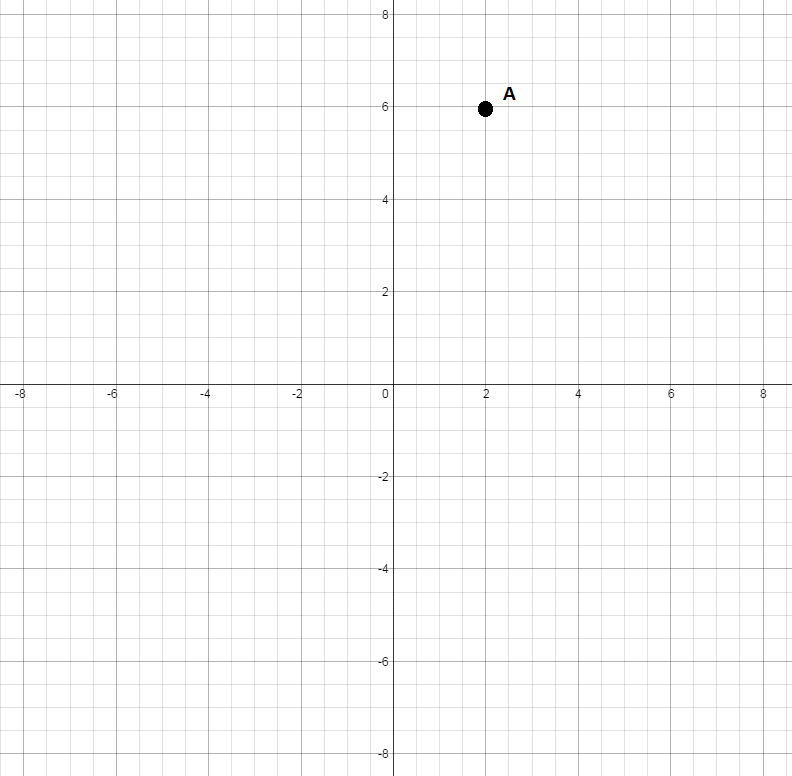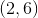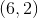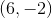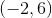Explanation:

Find the x-coordinate by going right on the horizontal x-axis until you come across the line that is directly under the point. The x-coordinate is.

Now, continue up until you reach the point and look across to the vertical y-axis. The y-coordinate is.are the coordinates for point A.

### Example Question #3 : How To Graph A Point

What are the coordinates for point C?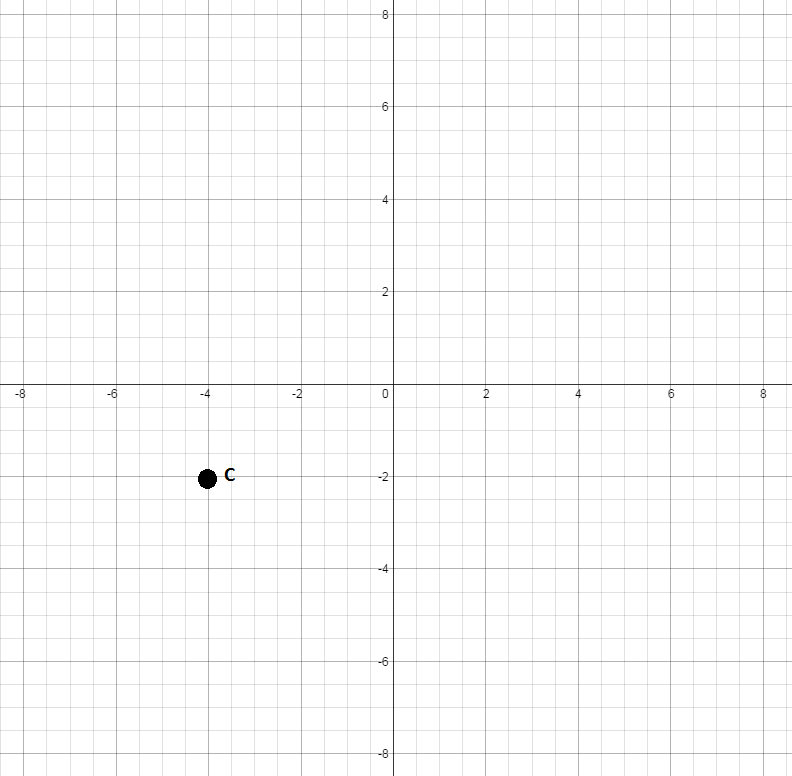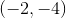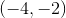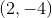Explanation:

Find the x-coordinate by going left on the horizontal x-axis until you come across the line that is directly above the point. The x-coordinate is.

Now, continue down until you reach the point and look across to the vertical y-axis. The y-coordinate is.are the coordinates for point C.

### Example Question #4 : How To Graph A Point

What are the coordinates for point D?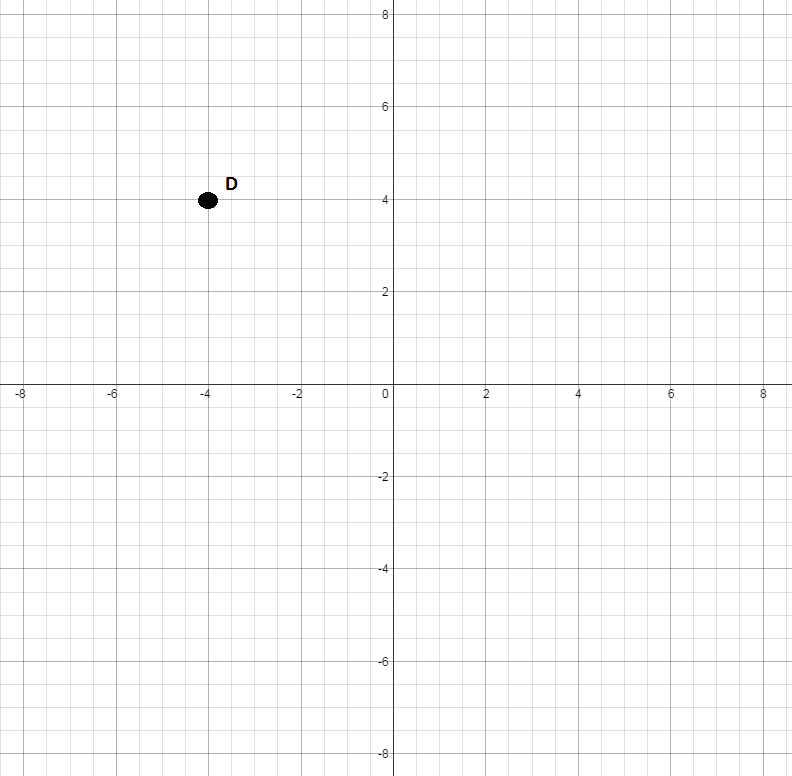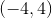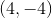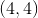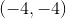Explanation:

Find the x-coordinate by going left on the horizontal x-axis until you come across the line that is directly under the point. The x-coordinate is.

Now, continue up until you reach the point and look across to the vertical y-axis. The y-coordinate is.are the coordinates for point D.

### Example Question #5 : How To Graph A Point

What are the coordinates for point E?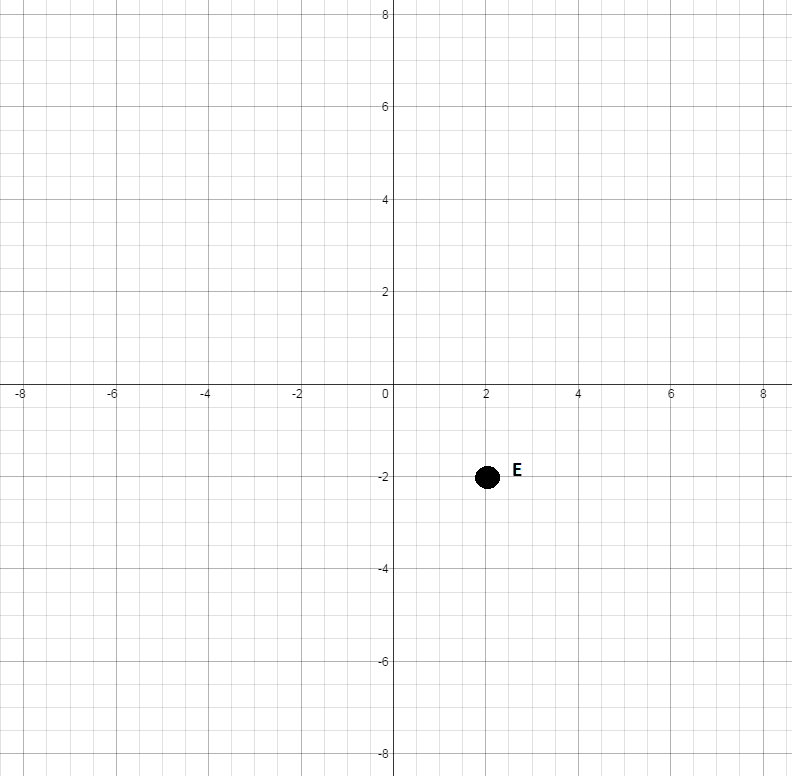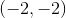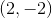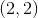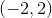Explanation:

Find the x-coordinate by going right on the horizontal x-axis until you come across the line that is directly above the point. The x-coordinate is.

Now, continue down until you reach the point and look across to the vertical y-axis. The y-coordinate is.are the coordinates for point E.

### Example Question #6 : How To Graph A Point

What are the coordinates for point F?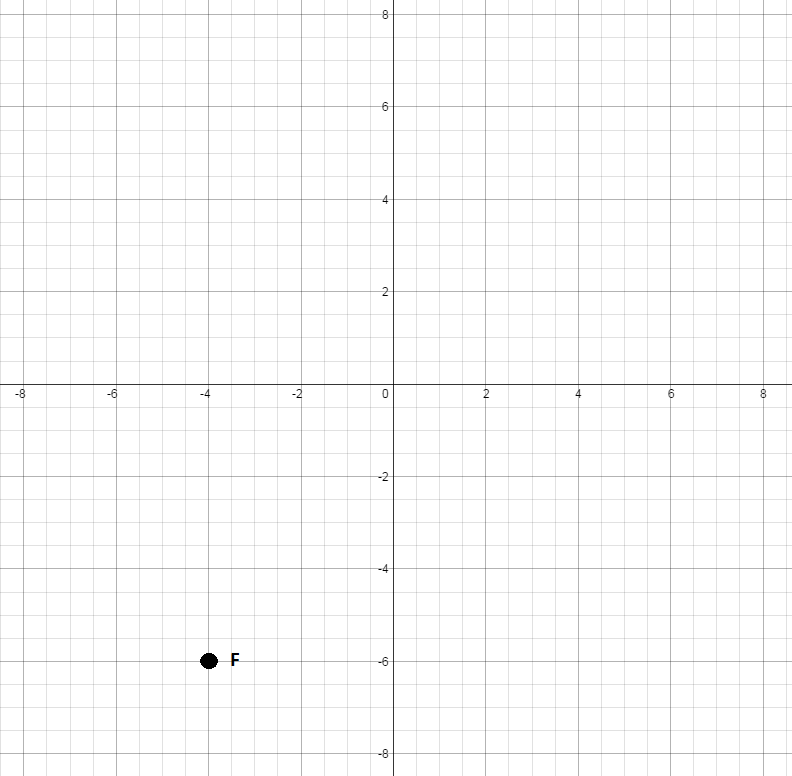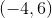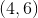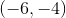Explanation:

Find the x-coordinate by going left on the horizontal x-axis until you come across the line that is directly above the point. The x-coordinate is.

Now, continue down until you reach the point and look across to the vertical y-axis. The y-coordinate is.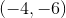are the coordinates for point F.

### Example Question #7 : How To Graph A Point

What are the coordinates for point G?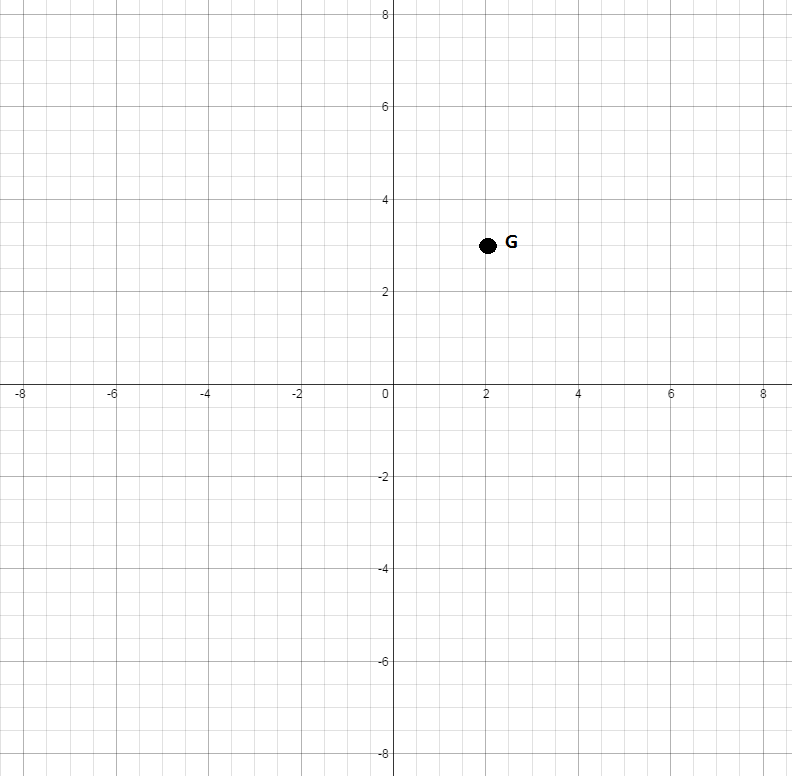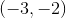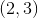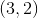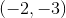Explanation:

Find the x-coordinate by going right on the horizontal x-axis until you come across the line that is directly under the point. The x-coordinate is.

Now, continue up until you reach the point and look across to the vertical y-axis. The y-coordinate is.are the coordinates for point G.

### Example Question #8 : How To Graph A Point

What are the coordinates for point H?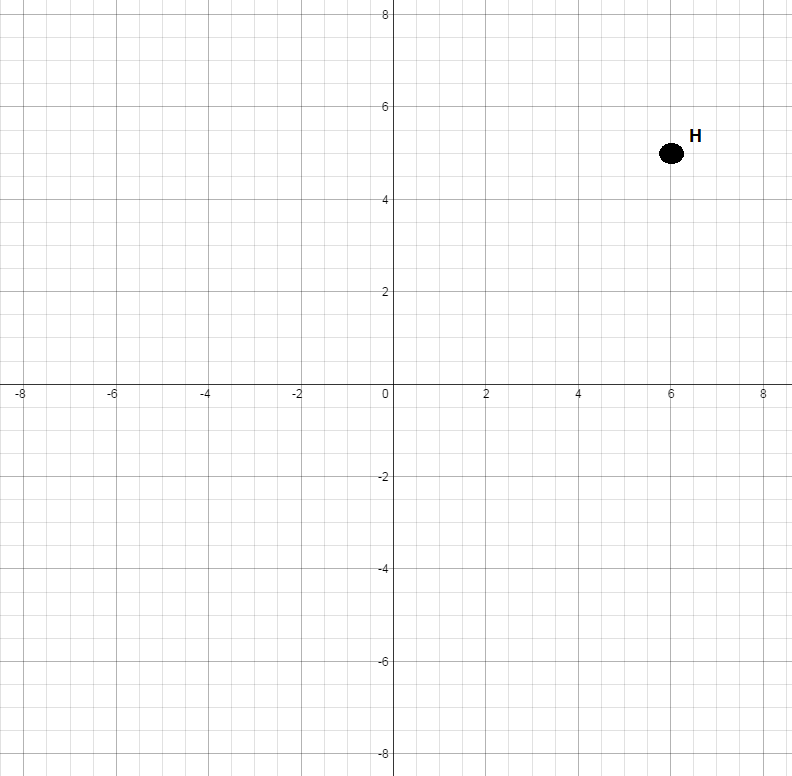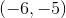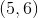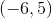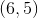Explanation:

Find the x-coordinate by going right on the horizontal x-axis until you come across the line that is directly under the point. The x-coordinate is.

Now, continue up until you reach the point and look across to the vertical y-axis. The y-coordinate is.are the coordinates for point H.

### Example Question #9 : How To Graph A Point

What are the coordinates for point J?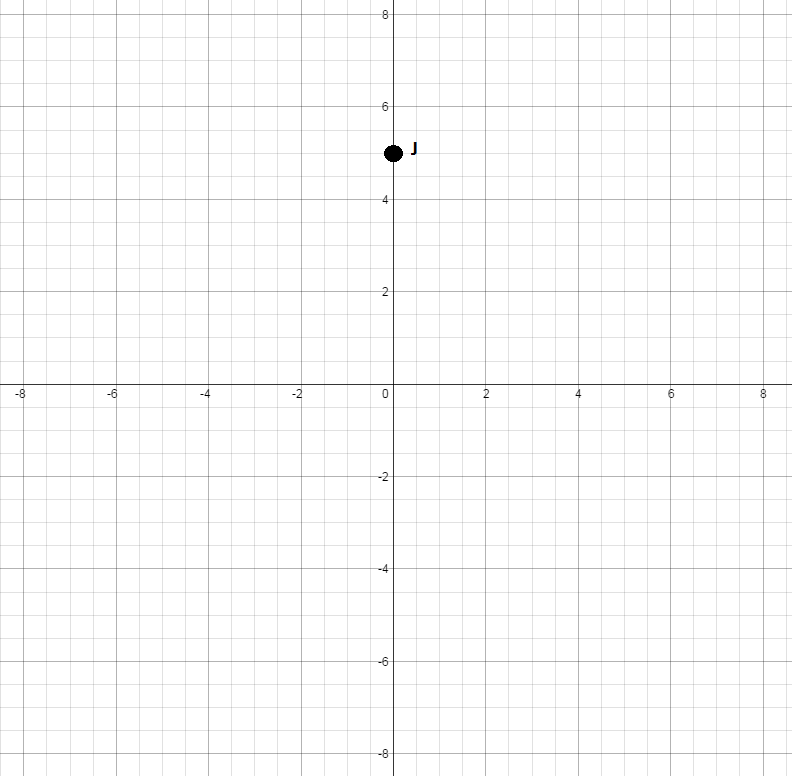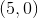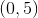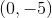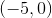Explanation:

Since there is no movement on the x-axis, the x-coordinate is.

Go up the vertical y-axis until you reach the point. The y-coordinate is.are the coordinates of point J.

### Example Question #1 : How To Graph A Point

What are the coordinates for point L?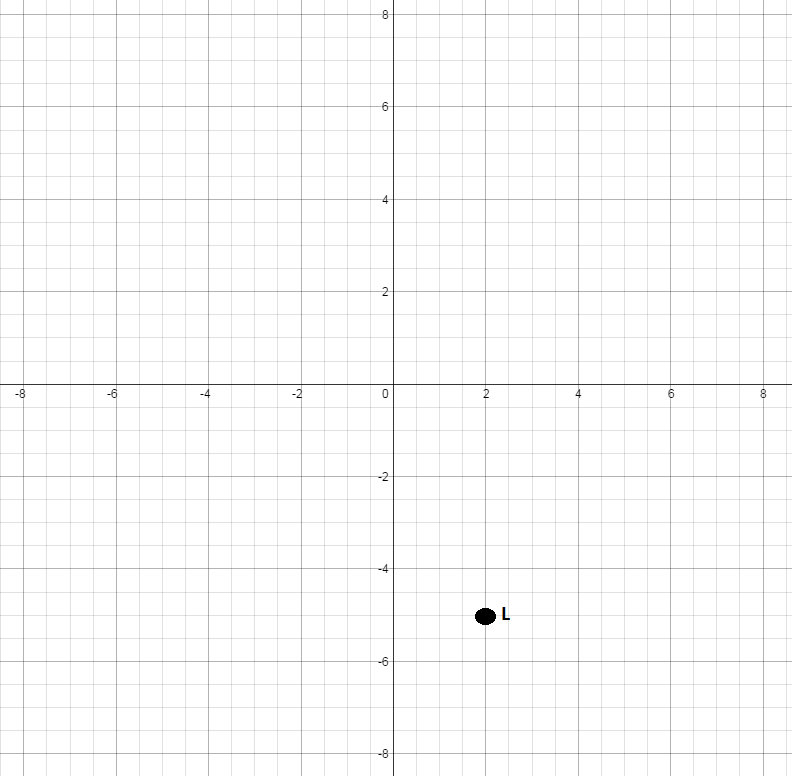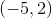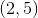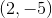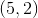Find the x-coordinate by going right on the horizontal x-axis until you come across the line that is directly above the point. The x-coordinate is.
Now, continue down until you reach the point and look across to the vertical y-axis. The y-coordinate is.are the coordinates for point L.